#### 期刊菜单

Research on Ships Trajectory Tracking Algorithm Based on Adaptive Control
DOI: 10.12677/DSC.2023.122009, PDF , HTML, XML, 下载: 130  浏览: 357

Abstract: This paper presents a fixed-time sliding mode control algorithm based on adaptive law for ships with disturbance. In the design of the controller, a dual layer nested adaptive scheme is used to reduce the chattering phenomenon, and the value of the control gain changes dynamically according to the external disturbance. Applying the fixed-time control theory to the research field of ships trajectory tracking can make the trajectory error independent of the initial state and reach the sliding surface in fixed time. In addition, the proposed control method can ensure the boundedness of the system trajectory. Finally, a simulation experiment is designed to verify the effectiveness and availability of the proposed method.

1. 引言

2. 船舶系统模型

2.1. 动力学模型

$\begin{array}{l}\stackrel{˙}{q}=J\left(\theta \right)\upsilon \\ M\stackrel{˙}{\upsilon }=-C\left(\upsilon \right)\upsilon -D\left(\upsilon \right)\upsilon +\tau +{d}_{1}\end{array}$ (1)

$J\left(\theta \right)=\left[\begin{array}{ccc}\mathrm{cos}\left(\theta \right)& -\mathrm{sin}\left(\theta \right)& 0\\ \mathrm{sin}\left(\theta \right)& \mathrm{cos}\left(\theta \right)& 0\\ 0& 0& 1\end{array}\right]$ $M=\left[\begin{array}{ccc}m-{X}_{\stackrel{˙}{u}}& 0& 0\\ 0& m-{Y}_{\stackrel{˙}{v}}& m{x}_{g}-{Y}_{\stackrel{˙}{r}}\\ 0& m{x}_{g}-{N}_{\stackrel{˙}{v}}& {I}_{z}-{N}_{\stackrel{˙}{r}}\end{array}\right]$

$C\left(\upsilon \right)=\left[\begin{array}{ccc}0& 0& {c}_{13}\left(\upsilon \right)\\ 0& 0& {c}_{23}\left(\upsilon \right)\\ -{c}_{13}\left(\upsilon \right)& -{c}_{23}\left(\upsilon \right)& 0\end{array}\right]$ $D\left(\upsilon \right)=\left[\begin{array}{ccc}{d}_{11}\left(\upsilon \right)& 0& 0\\ 0& {d}_{22}\left(\upsilon \right)& {d}_{23}\left(\upsilon \right)\\ 0& {d}_{32}\left(\upsilon \right)& {d}_{33}\left(\upsilon \right)\end{array}\right]$

${d}_{22}\left(\upsilon \right)=-{Y}_{v}-{Y}_{|v|v}|v|-{Y}_{|r|v}|r|$${d}_{23}\left(\upsilon \right)=-{Y}_{r}-{Y}_{|v|r}|v|-{Y}_{|r|r}|r|$${d}_{32}\left(\upsilon \right)=-{N}_{v}-{N}_{|v|v}|v|-{N}_{|r|v}|r|$${d}_{33}\left(\upsilon \right)=-{N}_{r}-{N}_{|v|r}|v|-{N}_{|r|r}|r|$ ，m表示船舶的质量， ${x}_{g}$ 表示船舶的重心到坐标系原点的距离。旋转矩阵 $J\left(\theta \right)$ 满足 ${J}^{\text{T}}\left(\theta \right)J\left(\theta \right)=I$$\stackrel{˙}{J}=JQ\left(r\right)$ 以及 $J\left(\theta \right)Q\left(r\right){J}^{\text{T}}\left(\theta \right)=Q\left(r\right)$ ，其中矩阵 $Q\left(r\right)$ 具有以下形式：

$Q\left(r\right)=\left[\begin{array}{ccc}0& -r& 0\\ r& 0& 0\\ 0& 0& 0\end{array}\right]$

$\begin{array}{c}{\stackrel{˙}{q}}_{2}=JQ\upsilon +J{M}^{-1}\left[-\left({C}_{0}\left(\upsilon \right)+\Delta C\left(\upsilon \right)\right)\upsilon -\left({D}_{0}\left(\upsilon \right)+\Delta D\left(\upsilon \right)\right)\upsilon +\tau +{d}_{1}\right]\\ =Q{q}_{2}-J{M}^{-1}\left({C}_{0}\left(\upsilon \right)+{D}_{0}\left(\upsilon \right)\right)\upsilon +J{M}^{-1}\tau +d\\ =f\left(q\right)+g\left(q\right)\tau +d\end{array}$ (2)

$\left\{\begin{array}{l}{\stackrel{˙}{q}}_{1}={q}_{2}\\ {\stackrel{˙}{q}}_{2}=f\left(q\right)+g\left(q\right)\tau +d\end{array}$ (3)

2.2. 轨迹误差模型

$\left\{\begin{array}{l}{\stackrel{˙}{q}}_{d1}={q}_{d2}\\ {\stackrel{˙}{q}}_{d2}={f}_{d}\left({q}_{d},{\tau }_{d}\right)\end{array}$ (4)

$\left\{\begin{array}{l}{\stackrel{˙}{x}}_{1}={x}_{2}\\ {\stackrel{˙}{x}}_{2}=f\left(q\right)+g\left(q\right)\tau +d-{f}_{d}\left({q}_{d},{\tau }_{d}\right)\end{array}$ (5)

3. 基于自适应的固定时间滑模控制算法

$s=c{x}_{1}+{x}_{2}$

$\tau \left(x\right)=-g{\left(q\right)}^{-1}\left[f\left(q\right)-{f}_{d}+c{x}_{2}+{k}_{1}\mathrm{sgn}\left(s\right)+{k}_{2}{‖s‖}^{\lambda }\mathrm{sgn}\left(s\right)+{k}_{3}{‖s‖}^{\mu }\mathrm{sgn}\left(s\right)+{k}_{4}s\right]$ (6)

$T\le \frac{1}{\left({k}_{1}-\kappa \right)/\sqrt{2}}+\frac{1}{{k}_{2}\left(\lambda -1\right){2}^{\frac{\lambda -1}{2}}}$ (7)

$\begin{array}{c}\stackrel{˙}{s}=f\left(q\right)+g\left(q\right)\tau +d-{f}_{d}+c{x}_{2}\\ =-\left({k}_{1}\mathrm{sgn}\left(s\right)+{k}_{2}{‖s‖}^{\lambda }\mathrm{sgn}\left(s\right)+{k}_{3}{‖s‖}^{\mu }\mathrm{sgn}\left(s\right)+{k}_{4}s\right)+d\end{array}$ (8)

$\begin{array}{c}\stackrel{˙}{V}=-{s}^{\text{T}}\left({k}_{1}\mathrm{sgn}\left(s\right)+{k}_{2}{‖s‖}^{\lambda }\mathrm{sgn}\left(s\right)+{k}_{3}{‖s‖}^{\mu }\mathrm{sgn}\left(s\right)+{k}_{4}s\right)+{s}^{\text{T}}d\\ \le -\left({k}_{1}-\kappa \right)s-{k}_{2}{‖s‖}^{\lambda +1}-{k}_{3}{‖s‖}^{\mu +1}-{k}_{4}{s}^{\text{T}}s\end{array}$ (9)

$\stackrel{˙}{V}\le -{2}^{\frac{1}{2}}\left({k}_{1}-\kappa \right){V}^{\frac{1}{2}}-{2}^{\frac{\lambda +1}{2}}{k}_{2}{V}^{\frac{\lambda +1}{2}}$ (10)

$T\le \frac{1}{\left({k}_{1}-\kappa \right)/\sqrt{2}}+\frac{1}{{k}_{2}\left(\lambda -1\right){2}^{\frac{\lambda -1}{2}}}$ (11)

${\stackrel{˙}{x}}_{1}={x}_{2}=-c{x}_{1}$ (12)

$\tau \left(x\right)=-g{\left(q\right)}^{-1}\left[f\left(q\right)-{f}_{d}+c{x}_{2}+k\left(t\right)\mathrm{sgn}\left(s\right)+{k}_{2}{‖s‖}^{\lambda }\mathrm{sgn}\left(s\right)+{k}_{3}{‖s‖}^{\mu }\mathrm{sgn}\left(s\right)+{k}_{4}s\right]$ (13)

$\tau ={\tau }_{1}+{\tau }_{2}$ ，其中

$\begin{array}{l}{\tau }_{1}=-g{\left(q\right)}^{-1}\left[f\left(q\right)-{f}_{d}+c{x}_{2}+{k}_{2}{‖s‖}^{\lambda }\mathrm{sgn}\left(s\right)+{k}_{3}{‖s‖}^{\mu }\mathrm{sgn}\left(s\right)+{k}_{4}s\right]\\ {\tau }_{2}=-g{\left(q\right)}^{-1}k\left(t\right)\mathrm{sgn}\left(s\right)\end{array}$ (14)

${\stackrel{˙}{\stackrel{¯}{\tau }}}_{eq}\left(t\right)=\frac{1}{\mathcal{l}}\left(-k\left(t\right)\mathrm{sgn}\left(s\right)-{\stackrel{¯}{\tau }}_{eq}\left(t\right)\right)$ (15)

$\frac{1}{\theta }‖{\stackrel{¯}{\tau }}_{eq}‖+\frac{\sigma }{2}>‖{\tau }_{eq}\left(t\right)‖=‖d\left(t\right)‖$ (16)

$\omega \left(t\right)=k\left(t\right)-\frac{1}{\theta }‖{\stackrel{¯}{\tau }}_{eq}‖-\sigma$ (17)

$\stackrel{˙}{k}\left(t\right)=-\epsilon \left(t\right)\mathrm{sgn}\left(\omega \left( t \right)\right)$

$\epsilon \left(t\right)={\nu }_{0}+\nu \left(t\right)$ (18)

$\beta \left(t\right)=\frac{h{\kappa }_{0}}{\theta }-\nu \left(t\right)$ (19)

$\stackrel{˙}{\nu }\left(t\right)=\alpha |\omega \left(t\right)|+{\nu }_{0}\sqrt{\alpha }\mathrm{sgn}\left(\beta \left(t\right)\right)$ (20)

${\stackrel{˙}{V}}_{0}=\omega \left(t\right)\stackrel{˙}{\omega }\left(t\right)+\frac{1}{\alpha }\beta \left(t\right)\stackrel{˙}{\beta }\left(t\right)$ (21)

$\begin{array}{c}\omega \left(t\right)\stackrel{˙}{\omega }\left(t\right)=\omega \left(t\right)\left(-\left({\nu }_{0}-\beta \left(t\right)+\frac{h{\kappa }_{0}}{\theta }\right)\mathrm{sgn}\left(\omega \left(t\right)\right)-\frac{1}{\theta }‖{\stackrel{˙}{\stackrel{¯}{\tau }}}_{eq}‖\right)\\ \le -{\nu }_{0}|\omega \left(t\right)|+\left(\beta \left(t\right)-\frac{h{\kappa }_{0}}{\theta }\right)|\omega \left(t\right)|+\frac{1}{\theta }|\omega \left(t\right)|{\stackrel{˙}{\stackrel{¯}{\tau }}}_{eq}\\ \le -{\nu }_{0}|\omega \left(t\right)|+\left(\beta \left(t\right)-\frac{h{\kappa }_{0}}{\theta }\right)|\omega \left(t\right)|+\frac{h{\kappa }_{0}}{\theta }|\omega \left(t\right)|\\ =-{\nu }_{0}|\omega \left(t\right)|+\beta \left(t\right)|\omega \left(t\right)|\end{array}$ (22)

$\beta \left(t\right)\stackrel{˙}{\beta }\left(t\right)=-\beta \left(t\right)\left(\alpha |\omega \left(t\right)|+{v}_{0}\sqrt{\alpha }\mathrm{sgn}\left(\beta \left(t\right)\right)\right)$ (23)

$\begin{array}{c}{\stackrel{˙}{V}}_{0}\le -{v}_{0}|\omega \left(t\right)|+\beta \left(t\right)|\omega \left(t\right)|-\frac{1}{\alpha }\beta \left(t\right)\left(\alpha |\omega \left(t\right)|+{v}_{0}\sqrt{\alpha }\mathrm{sgn}\left(\beta \left(t\right)\right)\right)\\ =-{v}_{0}|\omega \left(t\right)|-{v}_{0}\frac{1}{\sqrt{\alpha }}|\beta \left(t\right)|\\ \le -{v}_{0}\sqrt{{|\omega \left(t\right)|}^{2}+{\left(\frac{1}{\sqrt{\alpha }}|\beta \left(t\right)|\right)}^{2}}\\ =-{v}_{0}\sqrt{2{V}_{0}}\end{array}$ (24)

$\stackrel{˙}{v}\left(t\right)=\left\{\begin{array}{l}\alpha |\omega \left(t\right)|,\text{\hspace{0.17em}}\text{\hspace{0.17em}}|\omega \left(t\right)|>{\omega }_{0}\\ 0,\text{\hspace{0.17em}}\text{\hspace{0.17em}}\text{\hspace{0.17em}}\text{\hspace{0.17em}}\text{\hspace{0.17em}}\text{\hspace{0.17em}}\text{\hspace{0.17em}}\text{\hspace{0.17em}}\text{\hspace{0.17em}}\text{\hspace{0.17em}}\text{\hspace{0.17em}}\text{\hspace{0.17em}}\text{\hspace{0.17em}}\text{\hspace{0.17em}}其他\end{array}$ (25)

$\frac{1}{4}{\sigma }^{2}>{\omega }_{0}^{2}+\frac{1}{\alpha }{\left(\frac{h{\kappa }_{0}}{\theta }\right)}^{2}$ (26)

$|\omega \left(t\right)|>{\omega }_{0}$ 时， $\stackrel{˙}{v}\left(t\right)=\alpha |\omega \left(t\right)|$ 。对 $\beta \left(t\right)$ 进行求导，则有：

$\beta \left(t\right)\stackrel{˙}{\beta }\left(t\right)=-\beta \left(t\right)\nu \left(t\right)=-\alpha \beta \left(t\right)|\omega \left(t\right)|$ (27)

${\stackrel{˙}{V}}_{0}\le -{v}_{0}|\omega \left(t\right)|+\beta \left(t\right)|\omega \left(t\right)|+\frac{1}{\alpha }\left(-\alpha \beta \left(t\right)|\omega \left(t\right)|\right)=-{v}_{0}|\omega \left(t\right)|$ (28)

$|\omega \left(t\right)|\le {\omega }_{0}$ 时， $\stackrel{˙}{v}\left(t\right)=0$ 成立，于是有：

${\stackrel{˙}{V}}_{0}\le -{v}_{0}|\omega \left(t\right)|+\beta \left(t\right)|\omega \left(t\right)|$ (29)

${\stackrel{¯}{V}}_{0}=\left\{\left(\omega ,\beta \right):{V}_{0}<\frac{1}{2}{\omega }_{0}^{2}+\frac{1}{2\alpha }{\left(\frac{h{\kappa }_{0}}{\theta }\right)}^{2}\right\}$ (30)

$\begin{array}{c}{\int }_{0}^{+\infty }{\stackrel{˙}{V}}_{0}\text{d}t={V}_{0}\left(+\infty \right)-{V}_{0}\left(0\right)\\ =-{V}_{0}\left(0\right)\\ \le {\int }_{0}^{+\infty }-{v}_{0}|\omega \left(t\right)|\text{d}t\end{array}$ (31)

$\begin{array}{c}|k\left(t\right)|<|\omega \left(t\right)|+‖{\stackrel{¯}{\tau }}_{eq}\left(t\right)‖/\theta +\sigma \\ <|\omega \left(t\right)|+\left(1+{r}_{1}\right)\kappa /\theta +\sigma \end{array}$ (32)

$|\omega \left(t\right)|=|k\left(t\right)-‖{\stackrel{¯}{\tau }}_{eq}\left(t\right)‖/\theta -\sigma |<\sigma /2$

$k\left(t\right)>‖{\stackrel{¯}{\tau }}_{eq}\left(t\right)‖/\theta +\sigma /2>‖d\left(t\right)‖$ (33)

$|v\left(t\right)|=|h{\kappa }_{0}/\theta -\beta \left(t\right)|$ (34)

$|v\left(t\right)|<{\kappa }_{0}/\theta +|\beta \left(t\right)|$ (35)

4. 数值仿真

${q}_{d1}={\left[\mathrm{sin}\left(t\right)\text{\hspace{0.17em}}\text{\hspace{0.17em}}\mathrm{cos}\left(2t\right)\text{\hspace{0.17em}}\text{\hspace{0.17em}}2\mathrm{sin}\left(2t\right)\right]}^{\text{T}}$

${q}_{d2}={\left[\mathrm{cos}\left(t\right)\text{\hspace{0.17em}}\text{\hspace{0.17em}}-2\mathrm{sin}\left(2t\right)\text{\hspace{0.17em}}\text{\hspace{0.17em}}4\mathrm{cos}\left(2t\right)\right]}^{\text{T}}$

$c=\left[\begin{array}{ccc}1& 0& 0\\ 0& 1& 0\\ 0& 0& 1\end{array}\right]$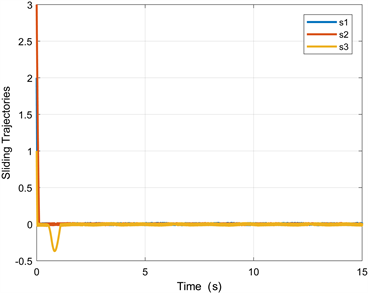Figure 1. Sliding mode variable under different initial states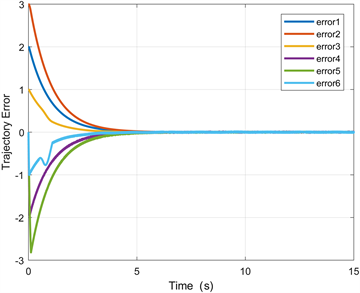Figure 2. Trajectory error under different initial states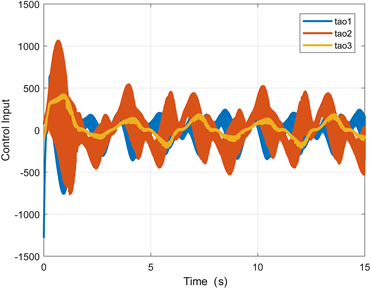Figure 3. Control input under adaptive gain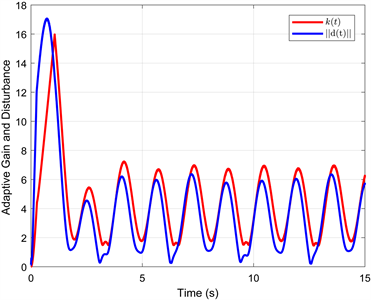Figure 4. Control gain and norm of disturbance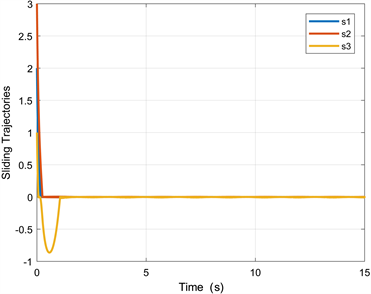Figure 5. Sliding mode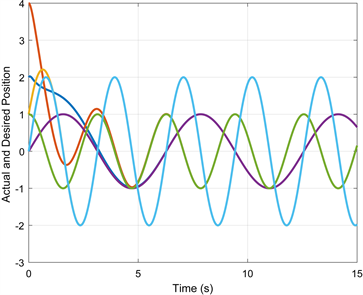Figure 6. The actual and desired trajectory of autonomous surface vehicle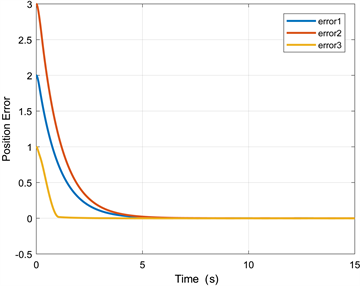Figure 7. The trajectory error of autonomous surface vehicle

5. 结论

  郑华荣, 魏艳, 瞿逢重. 水面无人艇研究现状[J]. 中国造船, 2020, 61(S1): 228-240.  李永杰, 张瑞, 魏慕恒, 等. 船舶自主航行关键技术研究现状与展望[J]. 中国舰船研究, 2021, 16(1): 32-44.  Li, L., Li, J. and Zhang, S. (2021) State-of-the-Art Trajectory Tracking of Autonomous Vehicles. Mechanical Sciences, 12, 419-432. https://doi.org/10.5194/ms-12-419-2021  Zhang, J., Zhang, Q. and Wang, Y. (2017) A New Design of Sliding Mode Control for Markovian Jump Systems Based on Stochastic Sliding Surface. Information Sciences, 391-392, 9-27. https://doi.org/10.1016/j.ins.2017.02.005  Mishra, R.N. and Mohanty, K.B. (2020) Development and Implementation of Induction Motor Drive Using Sliding-Mode Based Simplified Neuro-Fuzzy Control. Engineering Applications of Artificial Intelligence, 91, Article ID: 103593. https://doi.org/10.1016/j.engappai.2020.103593  Zhu, S., Polyakov, A. and Nakonechnyi, O. (2017) Sliding Mode Control Design for Linear Evolution Equations with Uncertain Measurements and Exogenous Perturbations. IFAC-PapersOnLine, 50, 8513-8517. https://doi.org/10.1016/j.ifacol.2017.08.872  Feng, J., Gao, S., Zhao, D., et al. (2020) Dynamic Output Feedback Sliding Mode Control for Non-Minimum Phase Systems with Application to an Inverted Pendulum. IFAC-PapersOnLine, 53, 5165-5170. https://doi.org/10.1016/j.ifacol.2020.12.1182  Liu, X., Zhang, M., Chen, J., et al. (2020) Trajectory Tracking with Quaternion-Based Attitude Representation for Autonomous Underwater Vehicle Based on Terminal Sliding Mode Control. Applied Ocean Research, 104, Article ID: 102342. https://doi.org/10.1016/j.apor.2020.102342  Boukadida, W., Benamor, A., Messaoud, H., et al. (2019) Multi-Objective Design of Optimal Higher Order Sliding Mode Control for Robust Tracking of 2-DoF Helicopter System Based on Metaheuristics. Aerospace Science and Technology, 91, 442-455. https://doi.org/10.1016/j.ast.2019.05.037  Zc, A., Tj, B. and Yn, A. (2020) Self-Triggered Sliding Mode Control for Digital Fly-by-Wire Aircraft System. Journal of the Franklin Institute, 357, 10492-10512. https://doi.org/10.1016/j.jfranklin.2020.08.028  Qureshi, M.S., Swarnkar, P. and Gupta, S. (2018) A Supervisory On-Line Tuned Fuzzy Logic Based Sliding Mode Control for Robotics: An Application to Surgical Robots. Robotics and Autonomous Systems, 109, 68-85. https://doi.org/10.1016/j.robot.2018.08.008  Chen, M., Wu, Q.X. and Cui, R.X. (2013) Terminal Sliding Mode Tracking Control for a Class of SISO Uncertain Nonlinear Systems. ISA Transactions, 52, 198-206. https://doi.org/10.1016/j.isatra.2012.09.009  Hao, C.A., St, A., Jh, A., et al. (2021) High-Order Sliding Mode Control of a Doubly Salient Permanent Magnet Machine Driving Marine Current Turbine. Journal of Ocean Engineering and Science, 6, 12-20. https://doi.org/10.1016/j.joes.2020.04.001  Hwang, C.-L. (1996) Sliding Mode Control Using Time-Varying Switching Gain and Boundary Layer for Electrohydraulic Position and Differential Pressure Control. Control Theory and Applications, IEE Proceedings, 143, 325-332. https://doi.org/10.1049/ip-cta:19960377  Zhang, L., Xia, Y., Shen, G., et al. (2021) Fixed-Time Attitude Tracking Control for Spacecraft Based on a Fixed-Time Extended State Observer. Science China Information Sciences, 64, 1-17. https://doi.org/10.1007/s11432-019-2823-9  Hu, C., Yu, J., Chen, Z., et al. (2017) Fixed-Time Stability of Dynamical Systems and Fixed-Time Synchronization of Coupled Discontinuous Neural Networks. Neural Networks, 89, 74-83. https://doi.org/10.1016/j.neunet.2017.02.001  Chen, C., Li, L., Peng, H., et al. (2020) A New Fixed-Time Stability Theorem and Its Application to the Fixed-Time Synchronization of Neural Networks. Neural Networks, 123, 412-419. https://doi.org/10.1016/j.neunet.2019.12.028  Wang, X., Guo, J., Tang, S., et al. (2019) Fixed-Time Disturbance Observer Based Fixed-Time Back-Stepping Control for an Air-Breathing Hypersonic Vehicle. ISA Transactions, 88, 233-245. https://doi.org/10.1016/j.isatra.2018.12.013